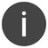# %IMPLIED

Convert an expression to zoned numeric implied-decimal

 WTSupported in traditional Synergy on Windows WNSupported in Synergy .NET on Windows USupported on UNIX VSupported on OpenVMS
```imp_dec_value = %IMPLIED(expression)
```

Return value

imp_dec_value

An implied-decimal value for the specified expression in zoned numeric representation. (d)

Arguments

expression

The expression to convert. (a or n)

Discussion

%IMPLIED converts an expression to an implied-decimal value in zoned numeric representation.

Expression is converted following the rules for moving data to an implied-decimal destination. (See Assignment statements for a description of these rules.) If expression is integer or decimal data type, the result won’t have any fractional precision. If expression is alpha, the fractional precision equals the number of digits to the right of the decimal point in expression.

Rounding and truncation come into effect if you have an alpha variable containing an implied-decimal value that has more than 28 places to the right of the decimal point.The use of %IMPLIED assumes that the called routine argument is IN only. If you use %IMPLIED to convert an n parameter to a d argument, you should explicitly modify the declared argument of the called routine to say “IN” if the direction is unspecified to allow the compiler to warn you if you write to the argument by mistake.

Examples

The following example uses alpha input as data for an implied-decimal arithmetic computation. Note that if the alpha variable contains any invalid hexadecimal characters, a “Bad digit encountered” error (\$ERR_DIGIT) is generated.

```subroutine math
a_avar              ,a
record
val1                ,d5.2
val2                ,d5.2
result              ,d10.2
proc
result = (val1*val2)/%implied(a_avar)
.
.
.
xreturn
endsubroutine
```